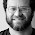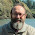## Thursday, March 21, 2013

### A must read: Edge Principal Components and Squash Clustering paper from Matsen and Evans

Had no idea this paper was coming out: PLOS ONE: Edge Principal Components and Squash Clustering: Using the Special Structure of Phylogenetic Placement Data for Sample Comparison.

Full citation: Matsen IV FA, Evans SN (2013) Edge Principal Components and Squash Clustering: Using the Special Structure of Phylogenetic Placement Data for Sample Comparison. PLoS ONE 8(3): e56859. doi:10.1371/journal.pone.0056859

And it is very very cool.  My lab has been working with / collaborating with / wanting to be like Erik Matsen for a few years now and this paper is one of the reasons why.  In this paper Matsen and Evans detail some really powerful and fascinating tools for phylogeny driven analysis of microbial communities.

Edge principal component analysis (edge PCA)
• "enables the detection of important differences between samples that contain closely related taxa".  (from the abstract)
• "applies the standard principal components construction to a “data matrix” generated from the differences between proportions of phylogenetic placements on either side of each internal edge of the reference phylogenetic tree." (from the Introduction)
Squash clustering:
•  "outputs a (rooted) clustering tree in which each internal node corresponds to an appropriate “average” of the original samples at the leaves below the node. Moreover, the length of an edge is a suitably defined distance between the averaged samples associated with the two incident nodes, rather than the less interpretable average of distances produced by UPGMA, the most widely used hierarchical clustering method in this context".  (from the Abstract)
• is hierarchical clustering with a novel way of merging clusters that incorporates information concerning how the data sit on the reference phylogenetic tree (from the Introduction)
Some nice figures tell the story of the methods pretty well:Figure 1. A graphical representation of the operation of edge principal components analysis (edge PCA). show more The phylogenetic distribution of reads for a given sample determines its position in the principal components projection. For the first axis, reads that fall below edges with positive coefficients on that axis' tree (marked in orange on the tree) move the corresponding sample point to the right, while reads that land on edges with negative coefficients (marked in green on the tree) move the corresponding sample point to the left. The second axis is labeled with a subtree of the first tree (the position of which is marked with a star on the first principal component tree): reads below edges with positive coefficients move sample points up, while reads below edges with negative coefficients move sample points down. The principal components shown here are the actual principal components for the example shown in Figures 4, 5, and 6.Figure 3. How the edge PCA algorithm works. show more (a) For every edge of the tree, the difference is taken between the number of reads on the non-root side the number of reads on the root side (root marked with a star). (b) The results of this are put into a matrix corresponding to the sample number (row) and the edge number (column). (c) The standard PCA algorithm is then applied, resulting in a collection of eigenvectors (the principal components) and eigenvalues. (d) These eigenvectors are indexed by the edges of the tree, and hence they can be mapped back onto the tree.Figure 2. A visual depiction of the squash clustering algorithm. When two clusters are merged, their mass distributions are combined according to a weighted average. The edges of the reference tree in this figure are thickened in proportion to the mass distribution (for simplicity, just a subtree of the reference tree is shown here). In this example, the lower mass distribution is an equal-proportion average of the upper two mass distributions. Similarities between mass distributions, such as the similarity seen between the two clusters for the G. vaginalis clade shown here, are what cause clusters to be merged. Such similarities between internal nodes can be visualized for the squash clustering algorithm; the software implementation produces such a visualization for every internal node of the clustering tree. Note that in this figure only the number of reads placed on each edge is shown, although each placement has an associated location on each edge when performing computation.

Anyway - check out the paper.  And follow their work - this is some pretty cool stuff.

UPDATE 3/21 - switched the captions for Figure 2 and 3 as per Matsen's comment that the legends were switched in production of the paper.

1.Hello Jonathan--

Wow, thank you for the very nice words and I'm glad that you like the paper.

I'm sorry to say that the images for Figures 2 and 3 were swapped (my fault). PLOS is working on correcting it, but it may take a while. In the mean time, perhaps I could make you some images to put on your blog with the right legends under the figures?

Thanks again,

Erick

1.Actually - I copied in the captions so I can fix them ... and they hopefully now read correctly (although now they are different than in the paper ...)

2.Hey, great! Thanks a bunch, Jonathan.

(Any ideas on how I can help along the process at PLOS? I may send girl scout cookies.)

Erick

1.no clue ...

3.[I have copied this comment of mine from Google+ here as well -- comments there do not seem to get replicated over here.]

Verry interrrrestink ... If you have a set of phenotypes which evolves on a tree by Brownian motion (not independent and not with equal variances) then the correct thing to do is to take the usual standardized phylogenetic contrasts -- they will be independent and identically distributed and their PCA analysis will be the correct one. But here the data are abundances in different environments, so the branch-by-branch statistics might make sense. There is some implicit model -- it would be nice to have insight into it.

It is also wonderful that some of species used in the analysis are of the genera Sneathia, named after an important microbial systematist and pioneer of clustering methods. And who was also a good guy to talk to, always interested in new methods. Peter Sneath would have loved this method.﻿

1.Thanks for copying -- I was about to but not sure of how to get that to happen automatically